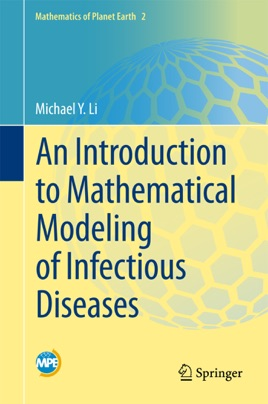• USD 39.99

## Descripción de editorial

This text provides essential modeling skills and methodology for the study of infectious diseases through a one-semester modeling course or directed individual studies.  The book includes mathematical descriptions of epidemiological concepts, and uses classic epidemic models to introduce different mathematical methods in model analysis.  Matlab codes are also included for numerical implementations.
It is primarily written for upper undergraduate and beginning graduate students in mathematical sciences who have an interest in mathematical modeling of infectious diseases.  Although written in a rigorous mathematical manner, the style is not unfriendly to non-mathematicians.